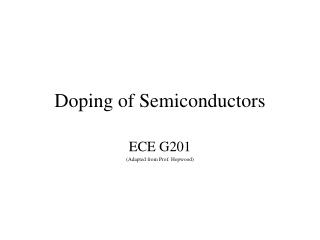DownloadDownload PresentationDoping of Semiconductors

# Doping of Semiconductors

Télécharger la présentation## Doping of Semiconductors

- - - - - - - - - - - - - - - - - - - - - - - - - - - E N D - - - - - - - - - - - - - - - - - - - - - - - - - - -
##### Presentation Transcript

1. Doping of Semiconductors ECE G201 (Adapted from Prof. Hopwood)

2. E(x) conduction band energy EC - - EV + + valence band energy x Reviewintrinsic semiconductor: no= po= ni -

3. The donor creates a small variation in the lattice potential resulting in an allowed state in the bandgap. E(x) Si conduction band - EC - P+ ED - EV valence band x n-type doping in siliconColumn V elements donate an electron to the conduction band

4. EC ED + EV What is the energy level, ED?We treat the ionized donor as a positive charge and consider the allowed energies of its extra valence electron using the Bohr model (an approximation!) E = Evac – mq2/2(4peonħ)2 = Evac – 13.6 eV/n2 But here the valence electron is free if E = EC. Also, the electron has an effective mass m* and the electron is in a semiconductor material with e = ereo. So, ED = EC – m*q2/2(4pereonħ)2 for Si, GaAs, Ge: er = 11.8, 13.2, 16 m*/me = 0.26, 0.067, 0.12

5. The lowest energy state (n=1) is most likely to be occupied, so… EC EC-ED ~ 13.6 eV(m*/me)(er)-2 < 0.05 eV This means that almost all donor atoms are ionized at room temperature since Et = kT = (8.63x10-5 eV/K)(300K) = 0.0259 eV and no ~ ND allowed ED + EV

6. Assumption: …the donor electron orbit (Y* Y) is big enough to encompass a large volume such that er represents the bulk material (not just a few atoms). This is not always the case (for example, when the effective mass is large). Then the actual donor energy levels are greater than this Bohr model calculation.

7. The acceptor creates a small variation in the lattice potential resulting in an allowed state in the bandgap. E(x) Si conduction band + EC B- EA - EV - valence band x p-type doping in siliconColumn III elements accept an electron from the valence band

8. EC - allowed EA EV Acceptor Energy Levels + typically, EA – EV < 0.05 eV

9. - - - - - - - - Summary, p-type Semiconductor EC Eg EA EV valence band with free holes po NA

10. - - - - - - - - Summary, n-type Semiconductor conduction band with free electrons no ND EC ED Eg EV

11. E - V+ EC=EP -qV EV Fe= -dEp/dx = -dEC/dx, Fe Electron Current Electrons move toward the positive potential (+) at a constant total energy (the kinetic energy increases but the potential energy decreases) until a collision with the an imperfection occurs. (EK0)

12. EC=EP -qV EV Fe= -dEp/dx = -dEC/dx = -qE “Band bending” E = (1/q)dEC/dx

13. Hole Current E - V+ EC=EP(x) -qV EV Fh= +dEp/dx, Fh

14. QUESTIONS?